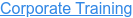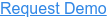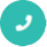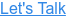#### Digital Marketing# Data Structures Interview Questions and Answers

by Mohammed, on Mar 19, 2018 12:30:33 PM## Q1. What is data structure?

Ans: A data structure is a way of organizing data that considers not only the items stored, but also their relationship to each other. Advance knowledge about the relationship between data items allows designing of efficient algorithms for the manipulation of data.

## Q2. List out few of the Application of tree data-structure?

Ans:

• The manipulation of Arithmetic expression
• Symbol Table construction
• Syntax analysis.

## Q3. What is a spanning Tree?

Ans: A spanning tree is a tree associated with a network. All the nodes of the graph appear on the tree once. A minimum spanning tree is a spanning tree organized so that the total edge weight between nodes is minimized.

## Q4. What is a linked list?

Ans: Linked list is a data structure which store same kind of data elements but not in continuous memory locations and size is not fixed. The linked lists are related logically.

## Q5. What is a node?

Ans: The data element of a linked list is called a node.

## Q6. What are priority queues?

Ans: A priority queue is a collection of elements such that each element has been assigned a priority.

## Q7. What is a string?

Ans: A sequential array of characters is called a string.

## Q8. What is Brute Force algorithm?

Ans: Algorithm used to search the contents by comparing each element of array is called Brute Force algorithm.

## Q9. What is the type of the algorithm used in solving the 8 Queens problem?

Ans: Backtracking.

## Q10. In RDBMS, what is the efficient data structure used in the internal storage representation?

Ans: B+ tree. Because in B+ tree, all the data is stored only in leaf nodes, that makes searching easier. This corresponds to the records that shall be stored in leaf nodes.

## Q11.  How can you overcome the limitations of arrays?

Ans: Limitations of arrays can be solved by using the linked list.

## Q12. What does node consist of?

Ans: Node consists of two fields:data field to store the element and link field to store the address of the next node.

## Q13. What is a queue ?

Ans: A Queue is a sequential organization of data. A queue is a first in first out type of data structure. An element is inserted at the last position and an element is always taken out from the first position.

## Q14. What are the types of Collision Resolution Techniques and the methods used in each of the type?

Ans: Open addressing (closed hashing),The methods used include:Overflow block.

## Q15. What are the methods available in storing sequential files?

Ans: Straight merging, Natural merging, Polyphase sort, Distribution of Initial runs.

## Q16. Mention some of the problem solving strategies?

Ans: The most widely strategies are listed below:

• Divide and conquer
• Binary doubling strategy
• Dynamic programming

## Q17. Minimum number of queues needed to implement the priority queue?

Ans: Two. One queue is used for actual storing of data and another for storing priorities.

## Q18. What are the notations used in Evaluation of Arithmetic Expressions using prefix and postfix forms?

Ans: Polish and Reverse Polish notations.

## Q19.  List out the areas in which data structures are applied extensively?

Ans: Compiler Design, Operating System, Database Management System, Statistical
analysis package, Numerical Analysis, Graphics, Artificial Intelligence, Simulation

## Q20. Translate infix expression into its equivalent post fix expression: (A-B)*(D/E)

Ans: (A-B)*(D/E) = [AB-]*[DE/] = AB-DE/*

## Q21. What are the limitations of arrays?

Ans:

• Arrays are of fixed size.
• Data elements are stored in continuous memory locations which may not be available always.
• Adding and removing of elements is problematic because of shifting the locations.

## Q22. What are the applications of binary tree?

Ans: Binary tree is used in data processing.

## Q23. What are the different types of traversing?

Ans: The different types of traversing are:

• Pre-order traversal-yields prefix from of expression.
• In-order traversal-yields infix form of expression.
• Post-order traversal-yields postfix from of expression.

## Q24. Define pre-order traversal?

Ans:

• Process the root node
• Process the left subtree
• Process the right subtree

## Q25. Define post-order traversal?

Ans:

• Process the left subtree
• Process the right subtree
• Process the root node

## Q26. Define in -order traversal?

Ans:

• Process the left subtree
• Process the root node
• Process the right subtree

## Q27. What actions are performed when a function is called?

Ans:

• Arguments are passed
• Local variables are allocated and initialized
• Transferring control to the function

## Q28. What is precision?

Ans: Precision refers the accuracy of the decimal portion of a value. Precision is the number of digits allowed after the decimal point.

## Q29. What is meant by sorting?

Ans: Ordering the data in an increasing or decreasing fashion according to some relationship among the data item is called sorting.

## Q30. What's the major distinction in between Storage structure and file structure and how?

Ans: The expression of an specific data structure inside memory of a computer system is termed storage structure in contrast to a storage structure expression in auxiliary memory is normally known as a file structure.

## Q31. What is divide and conquer method?

Ans: The basic idea is to divide the problem into several sub problems beyond which cannot be further subdivided. Then solve the sub problems efficiently and join then together to get the solution for the main problem.

## Q32. What is mean by d-queue?

Ans: D-queue stands for double ended queue. It is a abstract data structure that implements a queue for which elements can be added to front or rear and the elements can be removed from the rear or front. It is also called head-tail linked list

## Q33. What is AVL tree?

Ans: Avl tree is self binary tree in which balancing factor lie between the -1 to 1. It is also known as self balancing tree.

## Q34. What is binary tree?

Ans: Binary tree is a tree which has maximum no. of childrens either 0 or 1 or 2. i.e., there is at the most 2 branches in every node.

## Q35. What is the need for the header?

Ans: Header of the linked list is the first element in the list and it stores the number of elements in the list. It points to the first data element of the list.

## Q36. Define leaf?

Ans: In a directed tree any node which has out degree o is called a terminal node or a leaf.

## Q37. Stack can be described as a pointer. Explain?

Ans: Because stack will contain a head pointer which will always point to the top of the Stack.All Stack Operations are done using Head Pointer. Hence Stack ca be Described as a Pointer

## Q38. What do you mean by: Syntax Error, Logical Error, Run time Error?

Ans: Syntax Error-Syntax Error is due to lack of knowledge in a specific language. It is due to somebody does not know how to use the features of a language.We can know the errors at the time of compilation.
logical Error-It is due to the poor understanding of the requirement or problem.
Run time Error-The exceptions like divide a number by 0,overflow and underflow comes under this.

## Q39. What is the difference between a stack and a Queue?

Ans:

Stack: Represents the collection of elements in Last In First Out order. Operations includes testing null stack, finding the top element in the stack, removal of top most element and adding elements on the top of the stack.

Queue: Represents the collection of elements in First In First Out order.Operations include testing null queue, finding the next element, removal of elements and inserting the elements from the queue.
Insertion of elements is at the end of the queue.Deletion of elements is from the beginning of the queue

## Q40. What do you mean by overflow and underflow?

Ans: When new data is to be inserted into the data structure but there is no available space i.e.free storage list is empty this situation is called overflow.When we want to delete data from a data structure that is empty this situation is called underflow.

## Q41. Is it possible to find a loop in a Linked list?

Ans:

• Possilbe at O(n)
• Not possible
• Possible at O(n^2) only
• Depends on the position of loop

Solution: a. Possible at O(n)
Have two pointers say P1 and P2 pointing to the first node of the list.
Start a loop and Increment P1 once and P2 twice in each iteration. At any point of time if P1==P2 then there is a loop in that linked list. If P2 reaches NULL (end of linked list) then no loop exists.

## Q42. Two linked lists L1 and L2 intersects at a particular node N1 and from there all other nodes till the end are common. The length of the lists are not same. What are the possibilities to find N1?

Ans:

• Solution exist for certain cases only
• No linear solution exist
• Linear solution is possible
• Only Non-linear solution exist.

Solution: c. Linear solution is possible
Have two pointers say P1 pointing to the first node of L1 and P2 to that of L2. Traverse through both the lists. If P1 reaches L1’s last node, point it to the first node of L2 and continue traversing. Do the same thing for P2 when it reaches L2’s last node. (By doing this, we are balancing the difference in the length between the linked lists. The shorter one will get over soon and by redirecting to longer list’s head, it will traverse the extra nodes also.) Finally they will Meet at the Intersection node.

## Q43. void PrintTree (Tree T)

Ans:

{
if (T != NULL)
{
PrintTree (T-> Left);
PrintElement (T-> Element);
PrintTree (T->Right);
}
}
The above method ‘PrintTree’ results in which of the following traversal

• Inorder
• Preorder
• Postorder
• None of the above
Solution: a. Inorder
Inorder:
void PrintTree (Tree T)
{
if (T != NULL)
{
PrintTree (T-> Left);
PrintElement (T-> Element);
PrintTree (T->Right);
}
}
For preorder use this order
PrintElement (T-> Element);
PrintTree (T-> Left);
PrintTree (T->Right);
For postorder use this order
PrintTree (T-> Left);

PrintTree (T->Right);

PrintElement (T-> Element);

## Q44. Given a Binary Search Tree (BST), print its values in ascending order.

Ans:

• Perform Depth first traversal
• Perform Postorder traversal
• Perform Inorder traversal

Solution: d. Perform Inorder traversal
It is the properfy of BST and Inorder traversal.

## Q45. Is it possible to implement a queue using Linked List ?. Enqueue & Dequeue should be O(1).

Ans:

• Not possible to implement.
• Only Enqueue is possible at O(1).
• Only Dequeue is possible at O(1).
• Both Enqueue and Dequeue is possible at O(1)

Solution: d. Both Enqueue and Dequeue is possible at O(1)
Have two pointers H pointing to the Head and T pointing to the Tail of the linked list. Perform enqueue at T and perform dequeue at H. Update the pointers after each operations accordingly.

## Q46. Given a Tree, is it possible to find the greatest and least among leaves in linear time?

Ans:

• Solution depends on the tree structure
• Linear solution exist
• Only Non-linear solution exist.
• No linear solution exist

Solution: b. Linear solution exist
Have two variables Min and Max. Perform any tree traversal.Assign the first traversed leaf element to Min and Max for all other leaf elements check with these variables and update it accordingly. If a current element is < Min then update Min with that element. If it is > Min then check with Max.
Note: If you want to find the greatest and least among all nodes perform the checks for each node traversed.

## Q47. Is it possible to find find the greatest and least value among the nodes in a given BST without using any extra variables?

Ans:

• No solution exist.
• Solution need 2 extra variables
• Solution exist without any extra variables
• Solution need 1 extra variable

Solution:c Solution exist without any extra variables
As per BST property, the left most node should be the least one and the rightmost node should be the greatest. In other words, the first and last node of an Inorder traversal are the least and greatest among the nodes respectively.

## Q48. Is it possible to implement 2 stack in an array?

Ans: Condition: None of the stack should indicate an overflow until every slot of an array is used.

• Only 1 stack can be implemented for the given condition
• Stacks can not be implemented in array
• 2 stacks can be implemented for the given condition.
• 2 stacks can be implemented if the given condition is applied only for 1 stack.

Solution:c. 2 stacks can be implemented for the given condition
Start 1st stack from left (1st position of an array) and 2nd from right (last position say n). Move 1st stack towards right( i.e 1,2,3 ...n) and 2nd towards left (i.e n,n-1,n-2...1).

## Q49. Given two keys K1 & K2, write an algorithm to print all the elements between them with K1<=K2 in a BST.

Ans:

• Solution need 2 extra spaces
• Linear solution is possible without using any extra space
• No linear solution exist
• Solution need 1 extra space

Solution: Linear solution is possible without using any extra space
Perform an inorder traversal. Once you find K1 print it and continue traversal now, print all other traversed elements until you reach K2.
Note: If K1 == K2 stop once you find K1.

## Q50. How many stacks are required to implement a Queue?

Ans:

• One
• Two
• Three
• Two + one extra space.
Solution: Two
Have two stacks S1 and S2.
For Enqueue, perform push on S1.
For Dequeue, if S2 is empty pop all the elements from S1 and push it to S2. The last element you popped from S1 is an element to be dequeued. If S2 is not empty, then pop the top element in it.